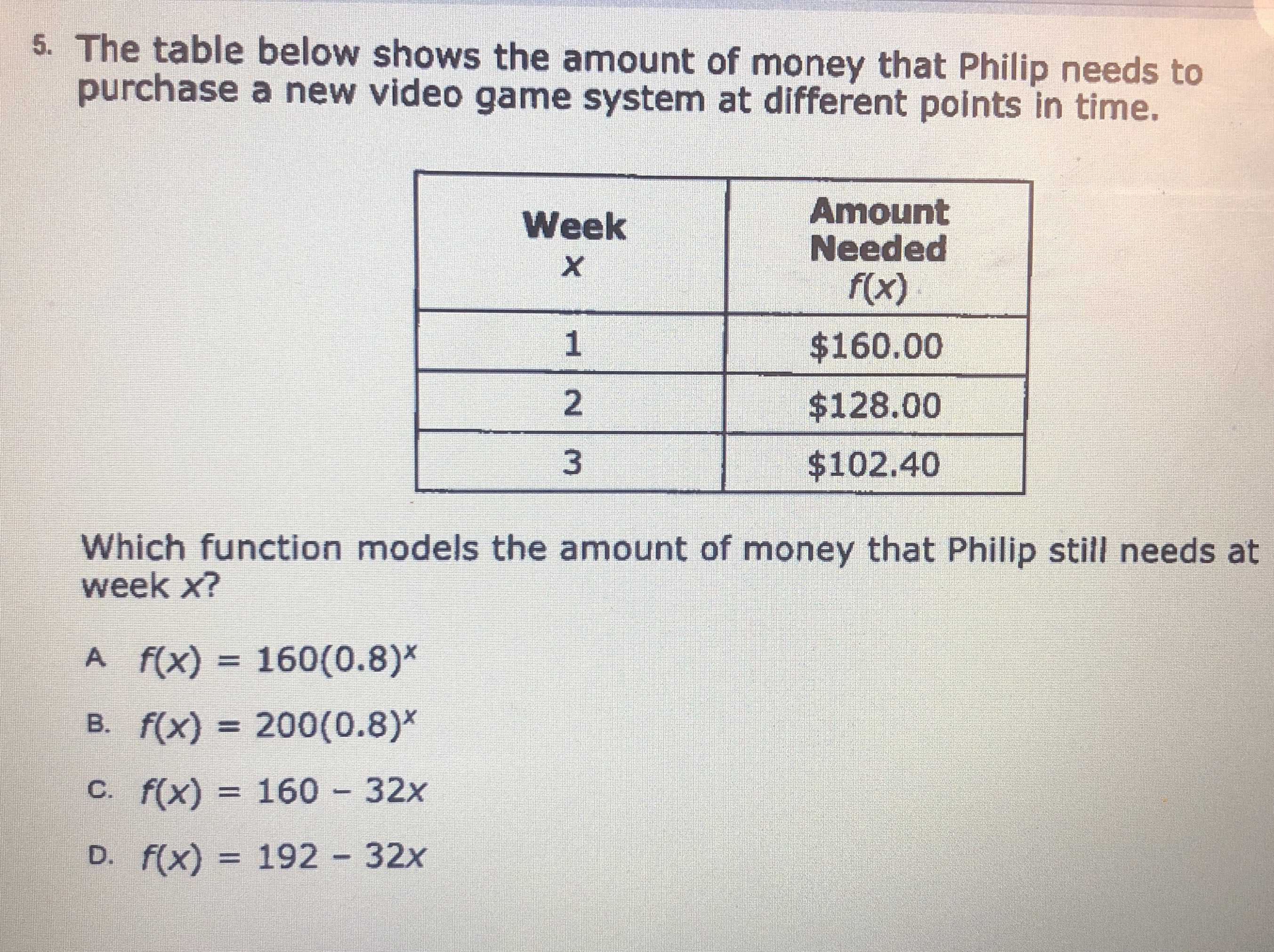### ¿Todavía tienes preguntas de matemáticas?

Pregunte a nuestros tutores expertos
Algebra
Pregunta5. The table below shows the amount of money that Philip needs to purchase a new video game system at different points in time.

Which function models the amount of money that Philip still needs at week $$x$$ ?

$$f ( x ) = 160 ( 0.8 ) ^ { x }$$

B. $$f ( x ) = 200 ( 0.8 ) ^ { x }$$

C. $$f ( x ) = 160 - 32 x$$

D. $$f ( x ) = 192 - 32 x$$